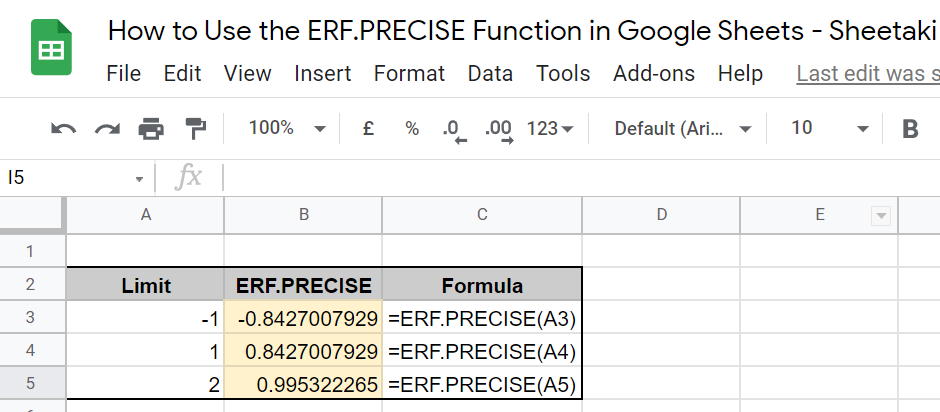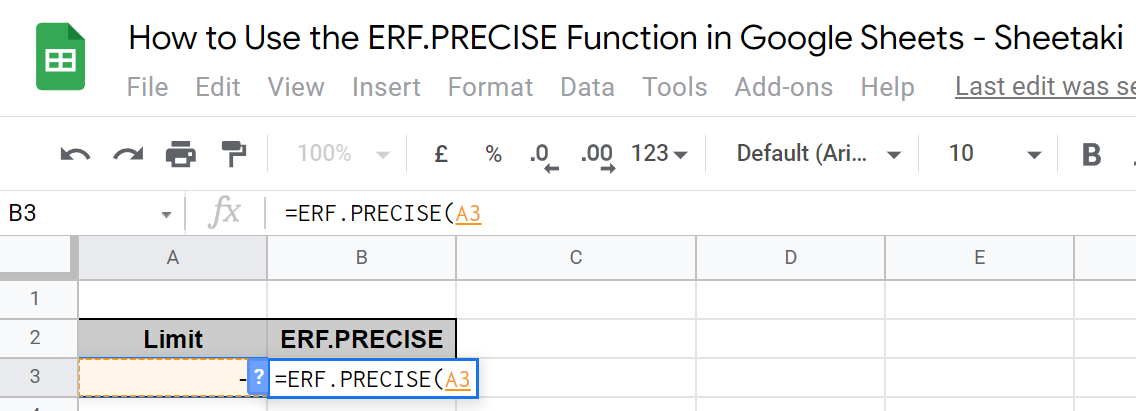How to Use the ERF.PRECISE Function in Google Sheets

The ERF.PRECISE function in Google Sheets calculates for the Gauss error function integrated between 0 and a given limit.

Just like the ERF function, the number generated represents how well your model fits the data and a lower number means you have less error. The main difference is that you can provide both upper and lower limits in the ERF function, while the ERF.PRECISE function necessitates 0 as one limit.

ERF.PRECISE is defined as follows:For a random variable normally distributed with a mean of 0 and a variance of 1/√2, ERF(x) describes the probability of the variable falling in the given range [0,x].

This basically works the same way as the ERF function, so check out our article on how to use the ERF function in Google Sheets to see if that’s a better use for you.

Let’s get to learning how to write the ERF.PRECISE function in Google Sheets.

The Anatomy of the ERF.PRECISE Function in Google Sheets

The syntax (the way we write) of the ERF.PRECISE function goes as follows:

=ERF.PRECISE(limit)

Let’s dissect this thing and understand what each of these terms means:

• = the equal sign is just how we start any function in Google Sheets.
• ERF.PRECISE () is our Gauss error function.
• limit is the lower or upper boundary for the integral.

Note that you will get the #VALUE! error value if your limit is non-numeric. Also, you can use both positive and negative integers as your given limit.

Real Example of Using the ERF.PRECISE Function in Google Sheets

Now, let’s take a look at some numbers I’m presenting to see how an ERT.PRECISE function will look like in Google Sheets.In the table, the function calculates the Gaussian error integral value for the limit as -1, then 1, and then 2. Besides the negative and positive signs, the result should be the same for (1,0) and (-1,0) since their distance on a distribution table is the same.

At this point, I’ll show you how you can do this yourself with comprehensive steps.

How to Use the ERF.PRECISE Function in Google Sheets

1. First, select the cell where you wish to put the result of your ERF.PRECISE function. In this case, that’s B3.2. Next, enter the equal sign ‘=’ to begin the function. Then, follow it with the name of the function, which is ‘ERF.PRECISE’ (or ‘erf.precise’, both should work). While typing that down, an auto-suggest box should appear with the different variations of the ERF function. Click on the ERF.PRECISE option.4. Proceed to enter the number that serves as your limit. In this case, we select the cell where the data is entered prior, which is A3.5. Then, simply enter a close parenthesis ‘)’ and select Enter on your keyboard.That’s it! You’ve just learned how to use the ERF.PRECISE function in Google Sheets.

What is the difference between ERF and ERF.PRECISE?

In an ERF function, you can provide both upper and lower limits. On the other hand, 0 is the default pair of your ERF.PRECISE limit. Note that an ERF function can technically serve as an ERF.PRECISE function since its upper_bound parameter is not mandatory.

What is ERFC.PRECISE?

ERFC stands for ‘complementary error function’ and gives out the value of 1.0 -erf(x).

ERFC.PRECISE calculated for the complementary error function integrated between a given lower limit and infinity.

How do I use ERF.PRECISE in Excel?

ERF.PRECISE is used the same in Google Sheets and Excel. Simply follow the instructions in this article to use the function in Excel. Note that while the ERF function has been available since before the 2010 update of Excel, that is not the same for ERF.PRECISE

You May Also LikeRemoving hyperlinks is useful when you want to remove texts automatically recognized by Google Sheets as hyperlinks. First,…How to Use YIELDMAT Function in Google Sheets

The YIELDMAT function in Google Sheets is useful to compute the yield of an interest-paying bond on maturity.…How to Use WEEKDAY Function in Google Sheets

The WEEKDAY function in Google Sheets is useful if you want to give a numerical value to a…How to Use the NORMDIST Function in Google Sheets

The NORMDIST function in Google Sheets is useful for finding out the value of the normal distribution function…